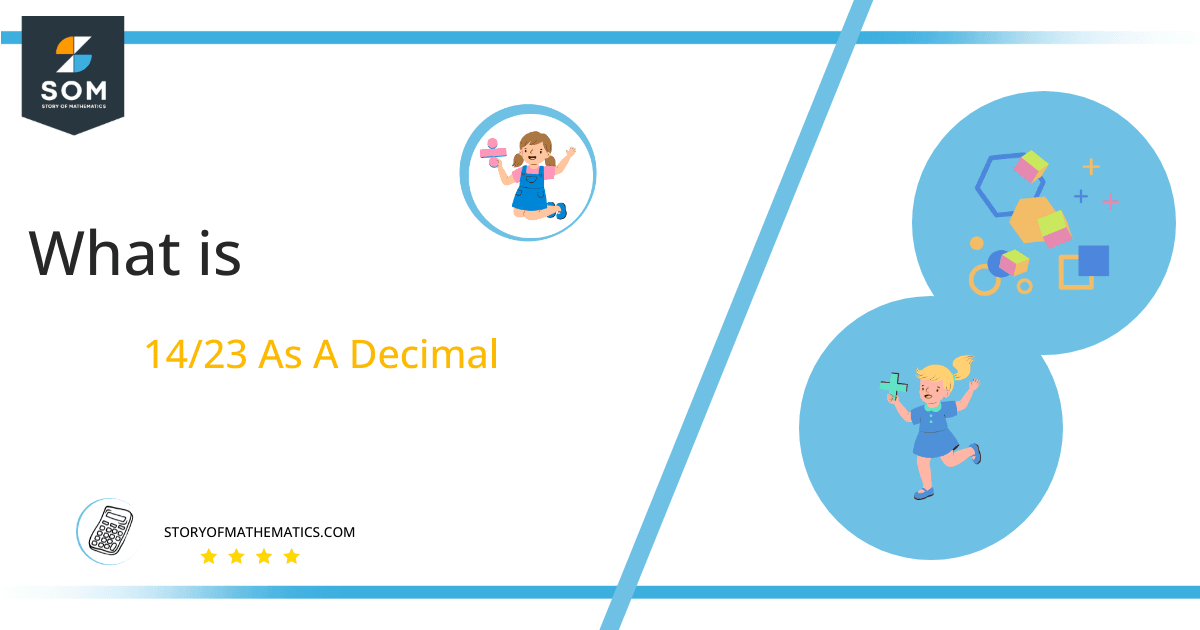# What Is 14/23 as a Decimal + Solution With Free Steps

The fraction 14/23 as a decimal is equal to 0.608.

If we divide a piece of pizza into different parts, all these parts are fractions of that whole pizza. In mathematics, this calculation is known as a fraction. It can be represented in the form of decimals.

Here, we are more interested in the division types that result in a Decimal value, as this can be expressed as a Fraction. We see fractions as a way of showing two numbers having the operation of Division between them that result in a value that lies between two Integers.Now, we introduce the method used to solve said fraction to decimal conversion, called Long Division, which we will discuss in detail moving forward. So, let’s go through the Solution of fraction 14/23.

## Solution

First, we convert the fraction components, i.e., the numerator and the denominator, and transform them into the division constituents, i.e., the Dividend and the Divisor, respectively.

This can be done as follows:

Dividend = 14

Divisor = 23

Now, we introduce the most important quantity in our division process: the Quotient. The value represents the Solution to our division and can be expressed as having the following relationship with the Division constituents:

Quotient = Dividend $\div$ Divisor = 14 $\div$ 23

This is when we go through the Long Division solution to our problem.Figure 1

## 14/23 Long Division Method

We start solving a problem using the Long Division Method by first taking apart the division’s components and comparing them. As we have 14 and 23, we can see how 14 is Smaller than 23, and to solve this division, we require that 14 be Bigger than 23.

This is done by multiplying the dividend by 10 and checking whether it is bigger than the divisor or not. If so, we calculate the Multiple of the divisor closest to the dividend and subtract it from the Dividend. This produces the Remainder, which we then use as the dividend later.

Now, we begin solving for our dividend 14, which after getting multiplied by 10 becomes 140.

We take this 140 and divide it by 23; this can be done as follows:

140 $\div$ 23 $\approx$ 6

Where:

23 x 6 = 138

This will lead to the generation of a Remainder equal to 140 – 138 = 2. Now this means we have to repeat the process by Converting the 2 into 20

Since if 2 is multiplied by 10 it becomes 20, which is still a smaller value than 23, we multiply 20 by 10 again to make it 200. For this, we add a zero in the quotient just after the decimal point. It makes 200 bigger than 23 and divisions are possible now.

Now we begin solving our dividend 200

200 $\div$ 23 $\approx$ 8

Where:

23 x 8 = 184

This, therefore, produces another Remainder which is equal to 200 – 184 = 16.

Finally, we have a Quotient generated after combining the three pieces of it as 0.608, with a Remainder equal to 16.Images/mathematical drawings are created with GeoGebra.